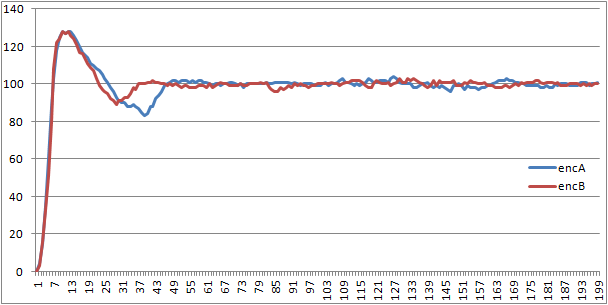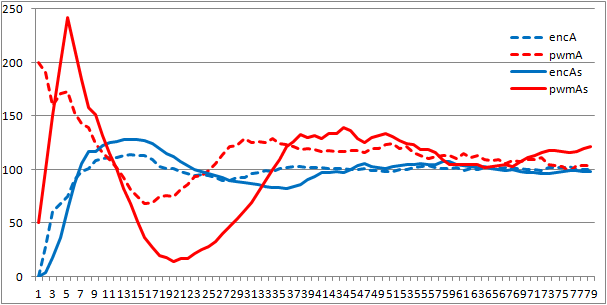# cr4cX Motor Controller

This blog is about the cr4's motor controller - updated 2012.03.22

Controller features:

• controls two H bridges;
• implements PID algorithm for controlling the speed of the motors;
• synchronize the motors;
• communicates through Serial and I2C/TWI;
• monitors the supply voltage;
• monitors the current through the motors.

Schematic (click to enlarge):I am using two Pololu motors with quadrature encoders. Each encoder have a resolution of 4288 ticks/output shaft rotation which means that for a wheel with 10 cm diameter the resolution is ~ 0,073 mm /encoder pulse.

The encoders are readed using the ATmega328 pin change interrupt.

PID and motor synchonization

I have tried some tunning methods for the PID algorithm but the problem was that the results without load were unusable in “real life”. I’ve did some timing tests and found that the I can output a few values through USART at 56kbps without interfering with the timings so I’ve built tunning/debugging logic in the firmware and a C++ application for PC to tune and debug the controller.

The recorded data is full of noise generated by my uneven floor so I was unable to do a precise tunning, however I was able to find some values that provided acceptable results for cr4 movement. The current tunning was done using an ugly brute force approach.

The PID function is called in a Timer2 interrupt function at 50Hz (tried at 100Hz but the discrete resolution of encoders did not give good results). Tunning is based of the error from the prescribed speed. Synchronization is done by accumulating the error for each motor and appling 1/16 of the difference to the current error, scalled by the speed of the motors. I should really try to change that procent.

Finally, I have implemented “limited acceleration” to the motors.

As a result, the maximum synchronization error between motors is 20 counts (uneven floor) which I consider it to be very good considering that a full rotation is 4288 counts.

Pure PWM driving, open-loop. In this figure is clearly seen the difference between the motorsPID with no synchronization between motorsPID with synchronization between motorsPID with synchronization between motors, limited acceleration, bigger overshoot and increased settle time (maybe I should tune D more) but reduced wear on the gears and drive trainPID with synchronization between motors, comparation for limited acceleration, the dotted values are without limited acceleration. Encoders and PWM values displayed.I am happy with the current results considering the 0,5% syncronization error but I may try other method in the future.

Analog values

Other

The software is not complete yet - I have to deal with maximum allowed current through the motors, minimum supply voltage for the motors and define a decent set of commands (only the test ones are implemented).

Software

The current version (21) must be cleaned a bit and some more tests need to be done however the PID code for one motor is:

`    if(usePID){        error = speedASP - encA - syncA;        iTermA += (error * ifA);        if(iTermA>IMAX) iTermA = IMAX;        if(iTermA<IMIN) iTermA = IMIN;        dt = lastEncA - encA;        lastEncA = encA;        res = error * pfA + iTermA + dt * dfA;        res += 64;    // SCALING_FACTOR/2        res /= 128;   // SCALING_FACTOR    }    else{        res = speedASP;    }    `then “res” is converted to PWM with limited acceleration using this:

`    if(res>=0){ // turn forward or backward        if(useSlowSteps){            if(moveTypeA==iMOVE_BACK) pwmA = 0 - res; // moveTypeA is correct beeing the new val            else                      pwmA = res;                        pwmA -= oldPwmA;                if(pwmA<minSlowStep) pwmA = minSlowStep;                if(pwmA>maxSlowStep) pwmA = maxSlowStep;            pwmA += oldPwmA;                        oldPwmA = pwmA;                        if(pwmA<0){ res = 0 - pwmA; pidMoveTypeA = iMOVE_BACK; }            else{       res = pwmA;     pidMoveTypeA = iMOVE_FWD;  }                        if(res>PWMax) res = PWMax; // trim res                        pwmA = 255 - res; // reverse the PWM value        }        else{            if(res>PWMax) res = PWMax; // trim res                        pwmA = 255 - res; // reverse the PWM value            pidMoveTypeA = moveTypeA;            if(pidMoveTypeA==iMOVE_BACK) oldPwmA = 0 - res;            else                         oldPwmA = res;        }    }    else{ // break        if(res<PWMin) res = PWMin; // trim res        pwmA = 255 + res;    // reverse the PWM value        pidMoveTypeA = iMOVE_BREAK;                oldPwmA = 0;    }`

where:

• speedASP is the prescribed speed for motor A;
• encA is the encoder counts since last calling interval for motor A;
• syncA is the synchronization error to be corrected in this call for motor A;
• pwmA is the PWM value to be sent to the motor A;
• IMAX and IMIN are the limits for PID’s integral term;
• minSlowStep and maxSlowStep are the limits for acceleration;
• due to construction, my drivers can “move” and “break” and the PWM is reverted, i.e. 0 is full speed and 255 is stop.

More to come…

I2C motor controller, this

I2C motor controller, this is something I was trying to do years ago and never quite did it nicely. I am not a strong programmer so I kind of gave up on tuning the PID and used a plain acceleration-top plate-deceleration method. Again, I’m going to follow your progress on this too.

PID tunning

The biggest challenge was the synchronization of the motors.

Regarding PID, when math is not the solution (I’ve got unusable results) remember that Brute Force never dies !

I’ve used brute force to find the P factor for a stable oscillation then derrived the P, I and D factors then I’ve adjusted them for a smaller overshoot (here is a link to the procedure).

Now I have to decide about the command set.